##### Pre-Calculus For Dummies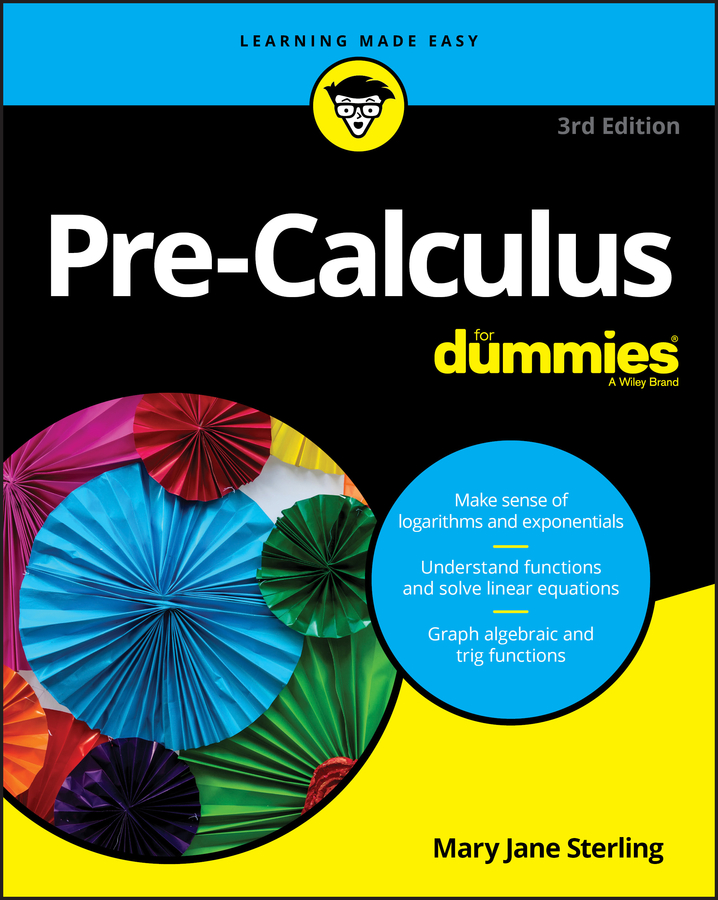Because a lot of pre-calculus work involves trigonometric functions, you need to understand ratios. One important ratio in right triangles is the tangent. The tangent of an angle theta, or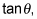is the ratio of the opposite leg to the adjacent leg. Here’s what it looks like in equation form: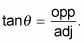Imagine for a moment that you’re an engineer. You’re working with a 39-foot tower with a wire attached to the top of it. The wire needs to attach to the ground and make an angle of 80 degrees with the ground to keep the tower from moving. Your task is to figure out how far from the base of the tower the wire should attach to the ground. Follow these steps:

1. Draw a diagram that represents the given information.

The figure shows the wire, the tower, and the known information.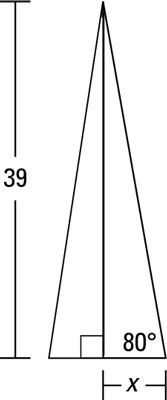2. Set up a trigonometric equation, using the information from the picture.

For this problem, you must set up the trigonometric equation that features tangent, because the opposite side is the length of the tower, the hypotenuse is the wire, and the adjacent side is what you need to find. You get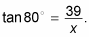3. Solve for the unknown.

Multiply both sides by the unknown x to get x tan 80 degrees = 39. Divide both sides by the tan 80 degrees to getSimplify to get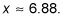The wire attaches to the ground about 6.88 feet from the base of the tower to form the 80-degree angle.